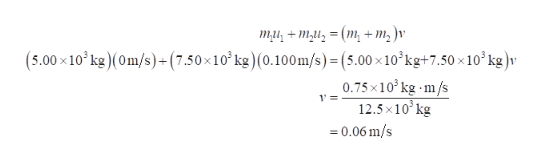# Two manned satellites approaching one another at a relative speed of 0.100 m/s intend to dock. The first has a mass of 5.00 ✕ 103 kg, and the second a mass of 7.50 ✕ 103 kg. If the two satellites collide elastically rather than dock, what is their final relative velocity? Adopt the reference frame in which the second satellite is initially at rest and assume that the positive direction is directed from the second satellite towards the first satellite. _________ m/s

Question
4 views

Two manned satellites approaching one another at a relative speed of 0.100 m/s intend to dock. The first has a mass of 5.00 ✕ 103 kg, and the second a mass of 7.50 ✕ 103 kg. If the two satellites collide elastically rather than dock, what is their final relative velocity? Adopt the reference frame in which the second satellite is initially at rest and assume that the positive direction is directed from the second satellite towards the first satellite.
_________ m/s

check_circle

Step 1

According to momentum conservation law, sum of initial velocities of satellite before collision will be equal to final velocity of system after collision. In...help_outlineImage Transcriptionclosemu,4m = (m, + m, )v (5.00x10 kg)(Om/s)+(7.50x10 kg) (0.100m/s) =(5.00 x 10'kg+7.50x10 kg)v 0.75x10 kg m/s 12.5x10 kg 0.06 m/s 1= fullscreen

### Want to see the full answer?

See Solution

#### Want to see this answer and more?

Solutions are written by subject experts who are available 24/7. Questions are typically answered within 1 hour.*

See Solution
*Response times may vary by subject and question.
Tagged in

### Physics